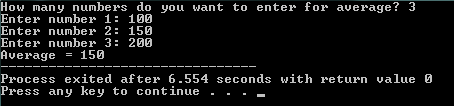# C: Arrays

#### Example: Array of Integers Created and Outputted in C

``````
#include

int main()
{
int ages = {29, 30, 18, 27};
int i;
for(i=0; i<4; ++i)
{
printf("Age: %d\n", ages[i]);
}

return 0;
}
``````

Output:

Age: 29
Age: 30
Age: 18
Age: 27

#### Example: Array used to Calculate Average

``````
#include
int main()
{
int temps, i, n, sum = 0, average;
printf("How many numbers do you want to enter for average? ");
scanf("%d", &n);
for (i=0; i < n; ++i)
{
printf("Enter number %d: ",i+1);
scanf("%d", &temps[i]);
sum += temps[i];
}
average = sum/n;
printf("Average = %d", average);
return 0;
}
``````

Output: